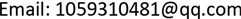MOSModeling and Simulation2324-8696Scientific Research Publishing10.12677/MOS.2018.73019MOS-26509MOS20180300000_34964721.pdf信息通讯 工程技术 基于联邦滤波器的多传感器故障检测隔离算法 Multi-Sensor Fault Detection and Isolation Algorithm Based on Federated Filter 薛伟罗晨12富占12启甲12null北京卫星信息工程研究所，北京060820180703153163© Copyright 2014 by authors and Scientific Research Publishing Inc. 2014This work is licensed under the Creative Commons Attribution International License (CC BY). http://creativecommons.org/licenses/by/4.0/Copyright © 2018 by authors and Hans Publishers Inc.1. 引言

2. INS/GNSS/视觉组合导航系统设计

2.1. 系统状态模型

X = [ ϕ e , ϕ n , ϕ u , δ v e , δ v n , δ v u , δ L , δ λ , δ h , ε b x , ε b y , ε b z , ε r x , ε r y , ε r z , ∇ x , ∇ y , ∇ z ] T (1)

X ˙ ( t ) = F ( t ) X ( t ) + G ( t ) W ( t ) (2)

2.2. 系统量测模型

Z G P S = H G P S X + V G P S (3)

SINS-视觉子系统量测方程中的量测信息由SINS输出的三维姿态、三维速度、三维位置分别与视觉导航系统输出的三维姿态、三维速度、三维位置的差值构成，三维姿态用， θ , φ , γ 来表示，分别为载体的俯仰角，偏航角及横滚角：

Z V i s i o n = H V i s i o n X + V V i s i o n (4)

V V i s i o n 为姿态、速度、位置测量误差白噪声矢量。

3. 残差c<sup>2</sup>-SPRT联合故障检测与隔离算法

3.1. 传感器故障模式

{ y = k ( t − t 0 )                                                                         线 性 故 障 y = k ( t − t 0 ) 2                                                       二 次 方 故 障 (5)

y = constant (6)

3.2. 残差c<sup>2</sup>算法

γ i ( k ) = Z i ( k ) − H i ( k ) X ^ i ( k / k − 1 ) (7)

X ^ i ( k / k − 1 ) = ϕ i ( k / k − 1 ) X ^ i ( k − 1 ) (8)

A i ( k ) = H i ( k ) P i ( k / k − 1 ) H i T ( k ) + R i ( k ) (9)

λ i ( k ) = γ i T ( k ) A i − 1 ( k ) γ i ( k ) (10)

λ i ( k ) 函数服从自由度为m的c2分布，即 λ i ( k ) ∼ χ 2 ( m ) ，m为观测矩阵 Z i ( k ) 的维数，故障判断准则为

{ λ i ( k ) > T D                                           有 故 障 λ i ( k ) ≤ T D                                           无 故 障 (11)

3.3. 改进SPRT故障检测算法

x ¯ k = 1 k ∑ i = 1 k x i (12)

σ k 2 = 1 k ∑ i = 1 k ( x i − x ¯ k ) 2 (13)

Detection threshold tabl
Pf\m12345678910
0.950.0040.100.350.711.141.632.172.733.323.94
0.500.461.392.373.364.355.356.357.348.349.34
0.201.643.224.645.997.298.569.8011.0312.2413.44
0.102.714.606.257.789.2410.6412.0213.3614.6815.99
0.053.845.997.829.4911.0712.5914.0715.5116.9218.31
0.016.649.2111.3413.2815.0916.8118.8420.0921.6723.21
0.00110.8313.8216.2718.4720.5222.4624.3226.1227.8829.59

3.4. 联合检测算法

1、残差c2检测输出1，SPRT检测输出1。两种检测算法均为检测出故障，判定系统工作正常，检测

2、残差c2检测输出0，SPRT检测输出0。两种检测算法均检测出故障，判定系统出现故障，检测结果3输出为0；

3、残差c2检测输出0，SPRT检测输出1。由于改进SPRT检测较残差c2检测，在故障出现时更为灵敏，且在故障消失时似然比需要一段时间恢复到阈值以下，因此这种情况几乎不会出现，理论上不计入讨论范围，实际出现时，按系统故障处理，检测结果3输出为0；

4、残差c2检测输出1，SPRT检测输出0。若为故障发生阶段，改进SPRT检测先于残差c2检测发现系统小量故障，该情况下，故障导致改进SPRT检测似然比增大，即当增量为正时，判定系统出现故障，检测结果3输出为0；若为故障消失阶段，改进SPRT检测似然比向阈值以下减小，即当增量为负时，判定系统工作正常，检测结果3输出为1。

4. 仿真与分析4.1. 仿真系统搭建

4.2. 仿真结果

5. 结束语